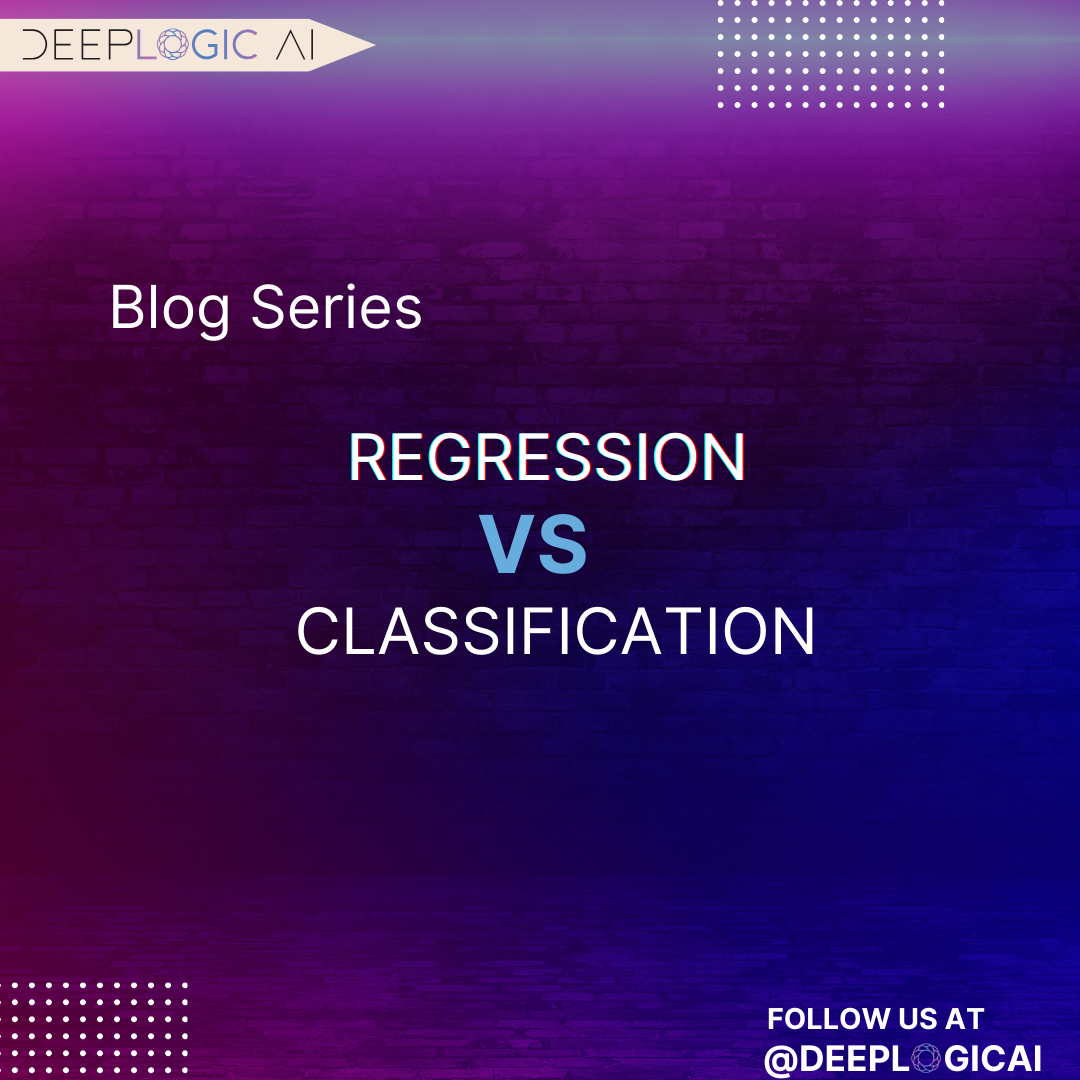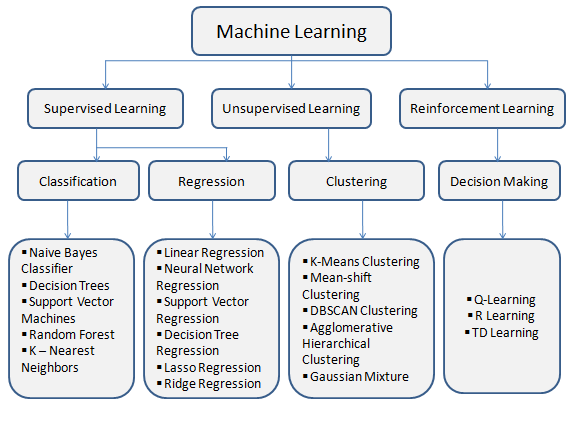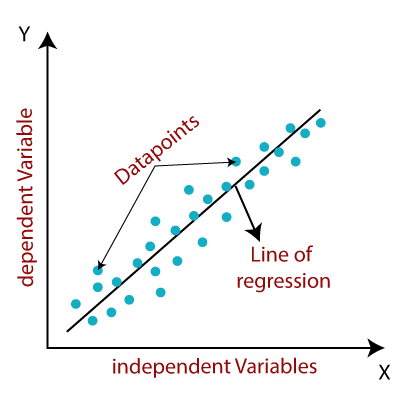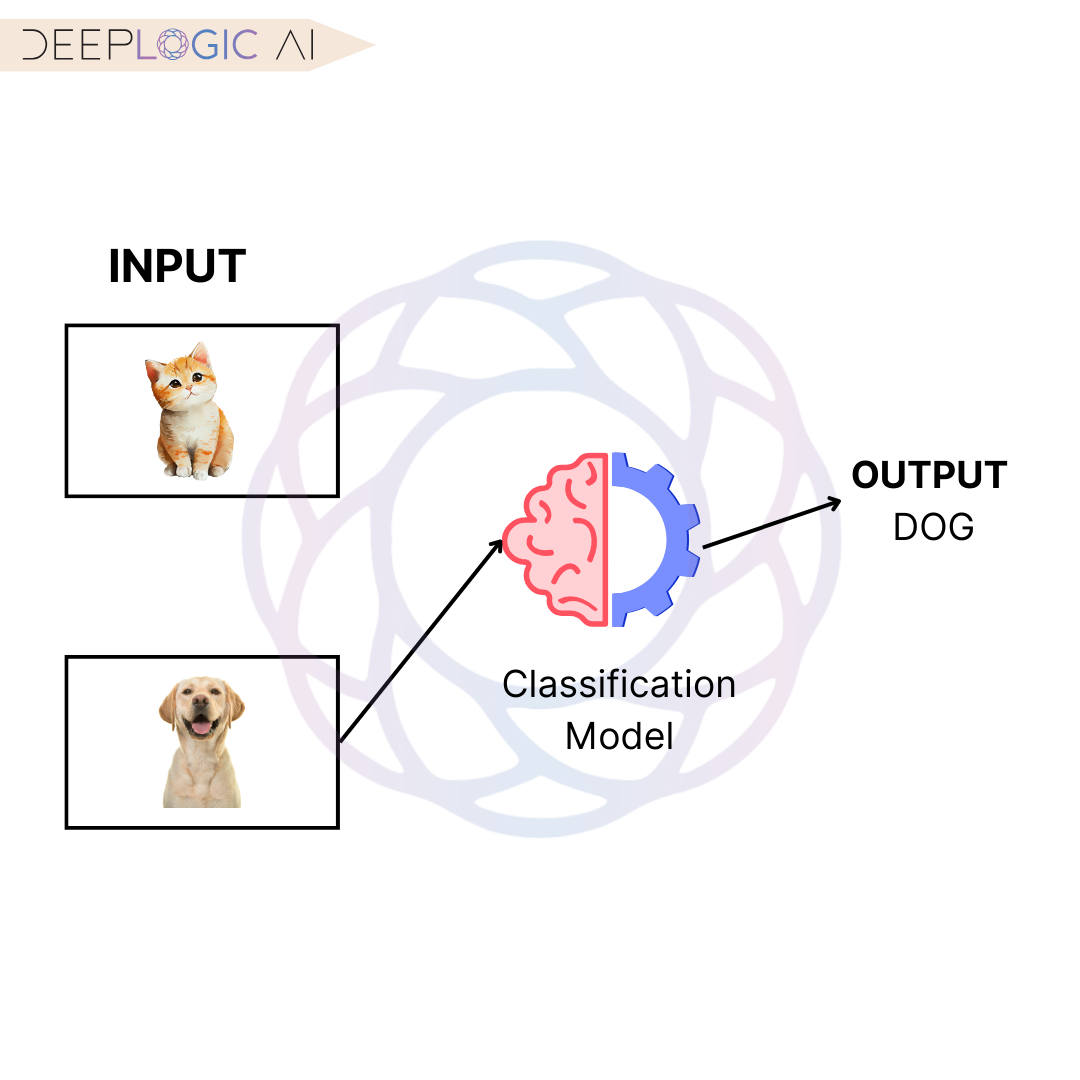## Regression VS ClassificationSupervised Learning

Supervised learning in machine learning encompasses a wide range of algorithms, each designed for specific tasks. Among these, regression and classification models are two fundamental approaches, each with its unique technical characteristics and applications. In this blog, we will delve into the technical details of these models, exploring how they work, their underlying mathematics, and their practical use cases.Image Source: Analytics Vidhya

Regression-Based Models

What is Regression?

Regression models are a cornerstone of supervised learning when the objective is to predict a continuous target variable based on independent features. At its core, regression seeks to establish a mathematical relationship between the input features and the continuous output variable.

How Regression Works ?

Let’s take simple linear regression model, the relationship between the features and the target variable is modeled as a linear equation

Y=ββ1ε

is the predicted output.

βis the y-intercept (the value of  when is zero).

βis the slope (the rate of change of with respect to X).

ε represents the error term, accounting for the noise in the data.

For more complex relationships, polynomial regression or other non-linear regression techniques can be employed. These models aim to find the best-fit line or curve that minimizes the sum of squared errors between the predicted values and the actual values.Image Source : IBM

1. Versatility: Regression models can capture linear and non-linear relationships, making them suitable for a wide range of applications.

2. Interpretability: Linear regression, in particular, offers straightforward interpretation of the relationship between input features and the target variable.

3. Quantitative Predictions: Regression models provide precise, quantitative predictions.

1. Sensitivity to Outliers:  Outliers in the data can significantly impact the model's performance.

2. Limited Handling of Categorical Data: Regression models require encoding categorical variables, which may introduce complexities.

3. Risk of Overfitting: Without appropriate regularization, regression models can overfit the training data.

Technical Evaluation Metrics

In regression, several technical metrics are used to evaluate model performance:

1. Mean Squared Error (MSE): Measures the average squared difference between predicted and actual values. It’s the most commonly used evaluation metric.

2. R-squared (R²): Indicates the proportion of the variance in the target variable explained by the model.

Practical Applications

Regression models are utilized in a variety of real-world applications, such as:

1. Stock Price Prediction: Forecasting stock prices based on historical data and market indicators.

2. Housing Price Prediction: Estimating house prices considering factors like location, size, and amenities.

3. Demand Forecasting: Predicting the demand for products to optimize inventory and production.

Classification-Based Models

What is Classification?

Classification models are employed when the goal is to predict a discrete target variable by categorizing data into specific classes or labels. The objective is to identify a decision boundary that effectively separates data points into predefined classes.

How Classification Works

Classification algorithms seek to create a decision boundary that separates the different classes in the target variable. The choice of the algorithm, such as Decision Trees, Random Forest, or Support Vector Machines, determines how this decision boundary is established.

1. Decision Trees: Divide the feature space into regions, creating a tree-like structure where each branch corresponds to a decision based on a feature.

2. Random Forest:  Ensembles multiple decision trees to improve accuracy and reduce overfitting.

3. Support Vector Machine (SVM): Finds the hyperplane that maximizes the margin between classes.1. Wide Applicability: Classification is versatile and applicable to various domains, including natural language processing and computer vision.

2. Robust Metrics: Classification models can be evaluated using metrics like Precision, Recall, and F1-Score to assess their performance.

1. Class Imbalance: Imbalanced datasets may lead to biased model performance.

2. Handling Noisy Data: Classification models may struggle when dealing with noisy or unstructured data.

3. Algorithm Sensitivity: The choice of classification algorithm significantly affects performance.

Technical Evaluation Metrics

In classification, technical metrics are essential for assessing model performance:

1. Precision: Measures the ratio of true positives to all predicted positives.

2. Recall: Indicates the ratio of true positives to all actual positives.

3. F1-Score: Combines precision and recall into a single metric.

Practical Applications

Classification models find applications in numerous areas, such as:

1. Spam Detection: Classifying emails as spam or not to filter unwanted messages.

2. Image Recognition: Identifying objects, people, or animals in images and videos.

3. Sentiment Analysis: Determining sentiment (positive, negative, neutral) in text data for market research and social media analysis.

Conclusion

Understanding the nuances of regression and classification models in supervised learning is crucial for making informed decisions in the development of AI solutions. These models offer distinct advantages and are suited to different types of tasks. By delving into the technical intricacies and selecting the most appropriate techniques, AI startups can design models tailored to the specific needs of their applications, driving innovation in the field of artificial intelligence.